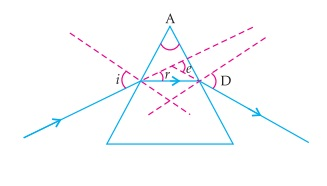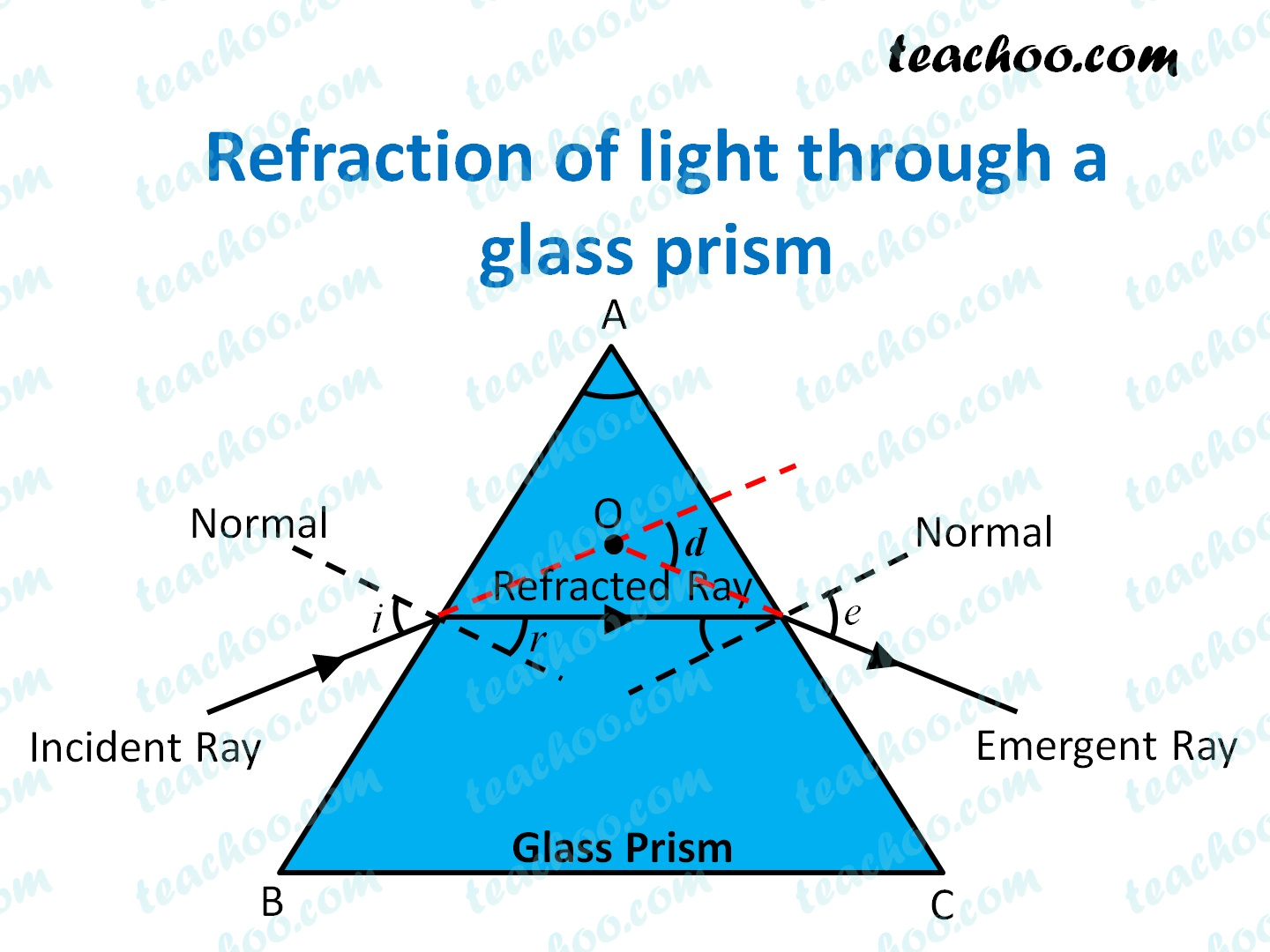MCQ from Past Year Papers

Class 10
Chapter 10 Class 10 - Light - Reflection and Refraction

##googletag.cmd.push(function() { googletag.display('div-gpt-ad-1669298377854-0'); });• Angle of incidence is the angle between incident ray and normal.
Here, angle of incidence is not with normal .   It is marked incorrectly

• Angle of refraction is the angle between refracted ray and normal.
So, here angle of incidence is angle r. So, it is marked correct

• Angle of emergence is the angle between emergent ray and normal.
Here, angle of emergence is not with normal .   It is marked incorrectly

• Angle of deviation is the angle between emergent ray and incident ray (when produced backwards).
So, here angle of incidence is angle D. So, it is marked correct

• Angle of prism is the vertex angle of the prism.
Here, it is angle A. It is marked correct

So, the correct answer is (b) -  ∠A and ∠D

Learn in your speed, with individual attention - Teachoo Maths 1-on-1 Class